• 以评价函数的方差值作为衡量调焦精度的标准，理论上计算了图像的信噪比对评价函数的期望和方差的影响，提出信噪比越高的图像中噪声越少，评价函数的方差值越小，调焦越准确。设计实验记录不同信噪比下，应用同样的...
• 对不同信噪比条件下条纹原理的阵列探测器获得的激光雷达回波实验信号的测绘误差进行了分析并获得了激光回波信噪比对激光雷达测绘精度影响的实验规律，并对该种探测系统的背景噪声特性进行了研究，在此基础上建立了...
• ## 信噪比

万次阅读 2016-01-25 17:51:27
看到很多朋友都在谈论信噪比这个概念，正好前段时间学到了这个，特此拿出来班门弄斧一下。  　信噪比--SNR(有时写成S/N)是signal-to-noise ratio的缩写，是指有用信号(规定输入电压下的输出信号电压)和噪声信号...

fft原理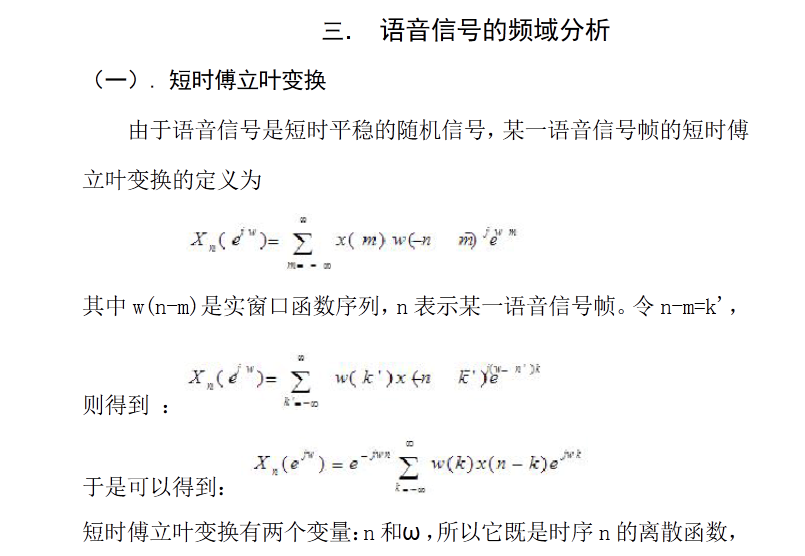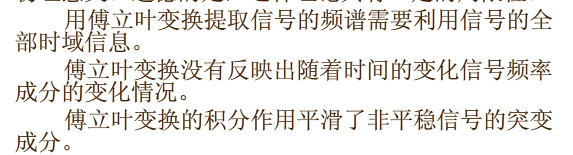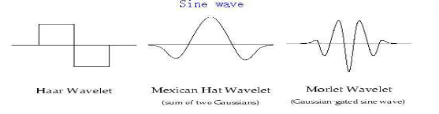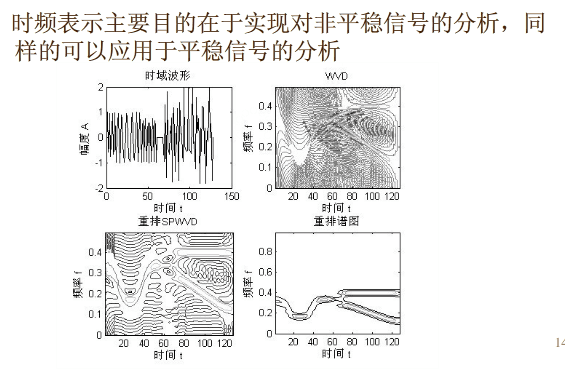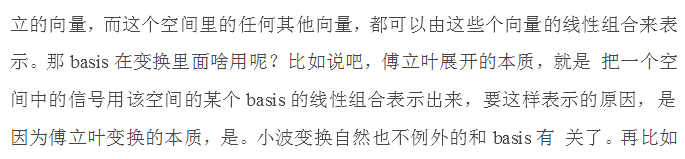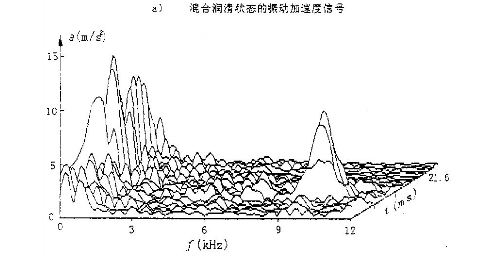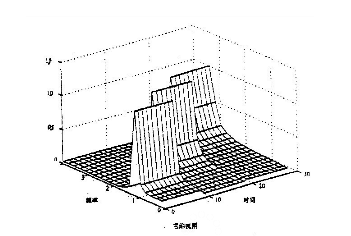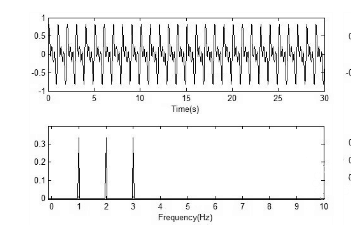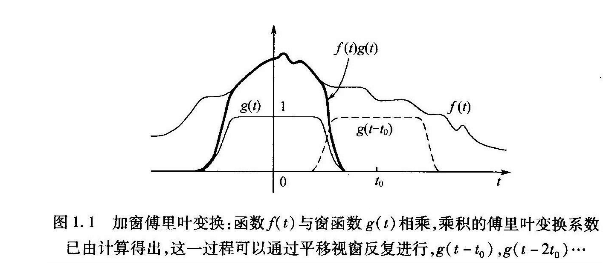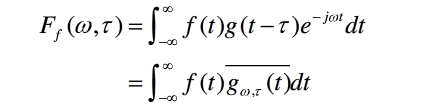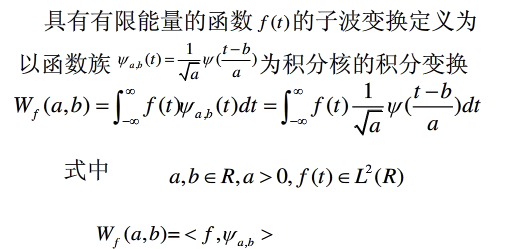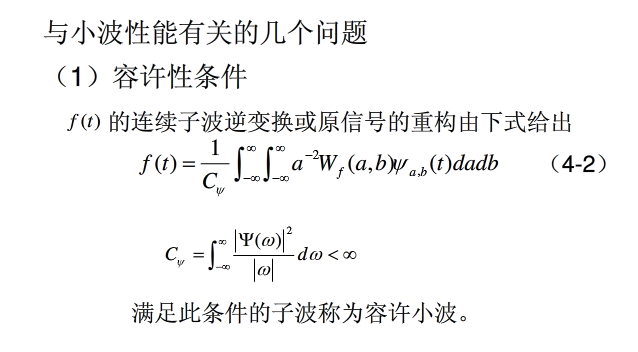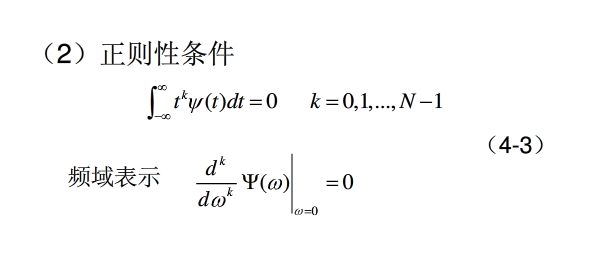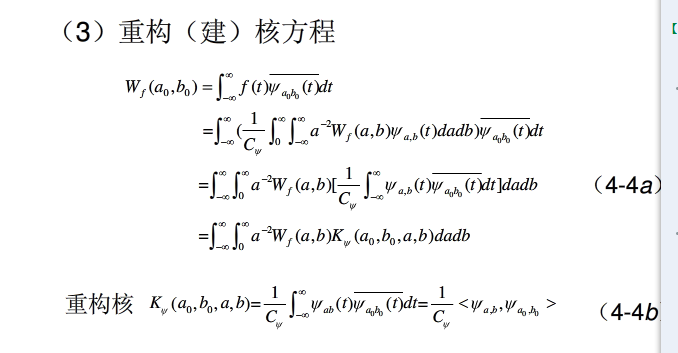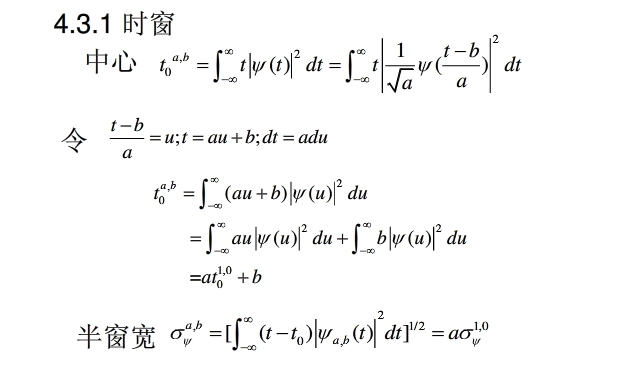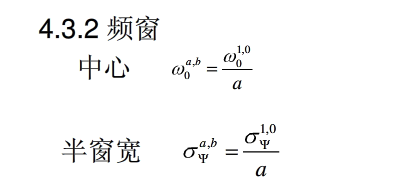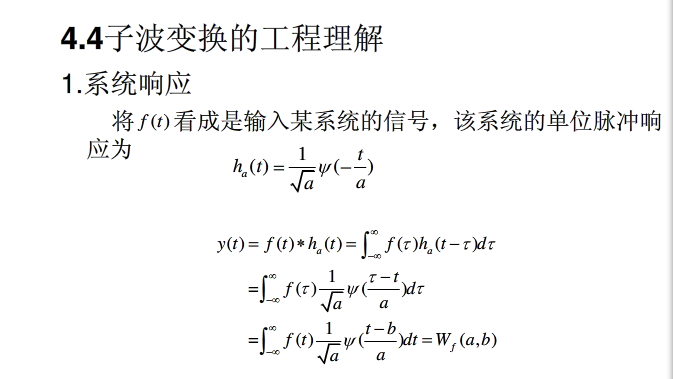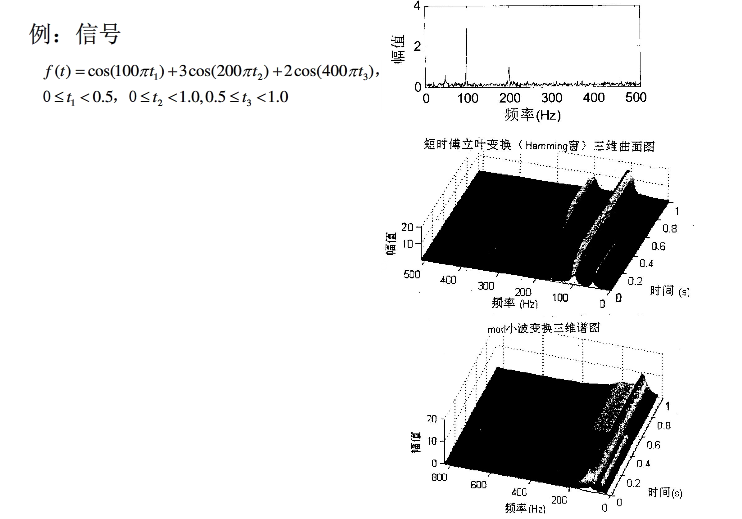能量谱
（5）能量信号频谱通常既含有幅度也含有相位信息；幅度谱的平方（二次量纲）又叫能量谱（密度），它描述了信号能量的频域分布；功率信号的功率谱（密度）描述了信号功率随频率的分布特点（密度：单位频率上的功率），业已证明，平稳信号功率谱密度恰好是其自相关函数的傅氏变换。对于非平稳信号，其自相关函数的时间平均（对时间积分，随时变性消失而再次退变成一维函数）与功率谱密度仍是傅氏变换对；
滤波器
LTI滤波器结构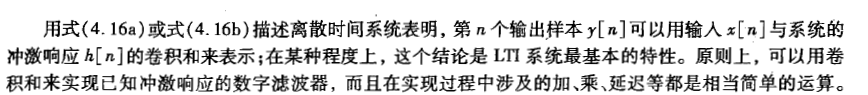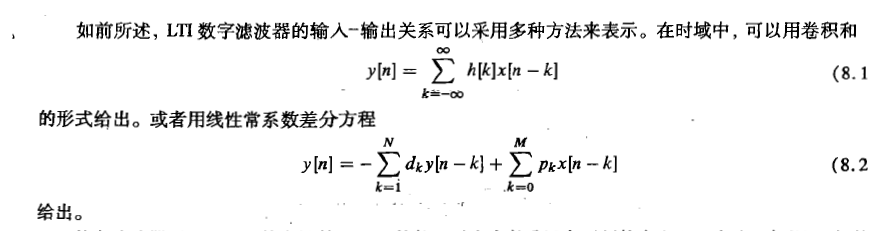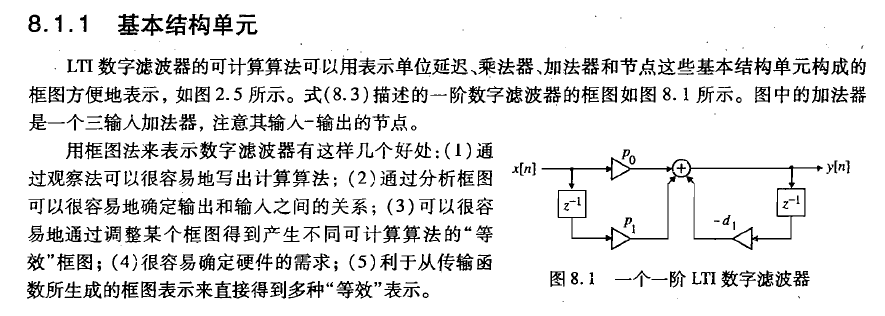fir滤波器结构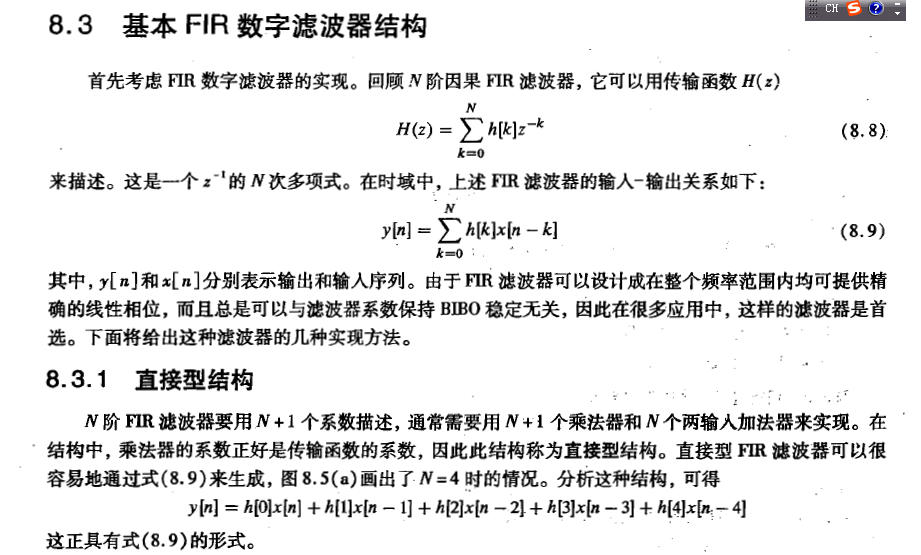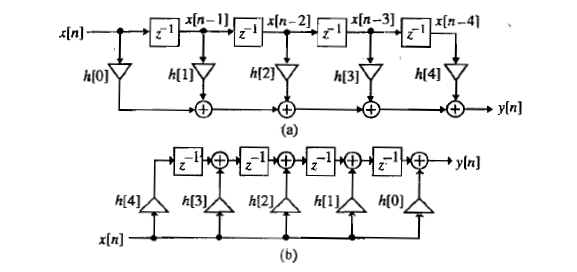IIR结构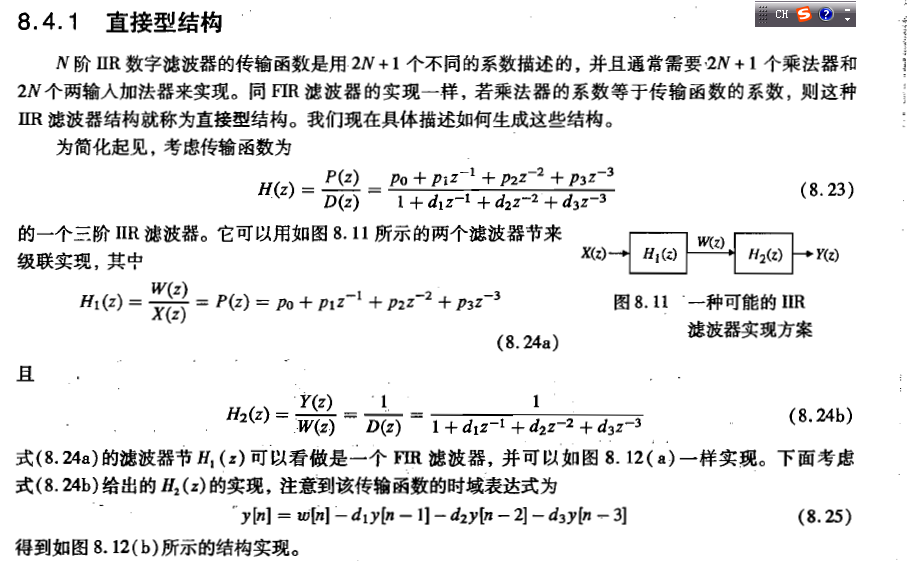切比雪夫滤波器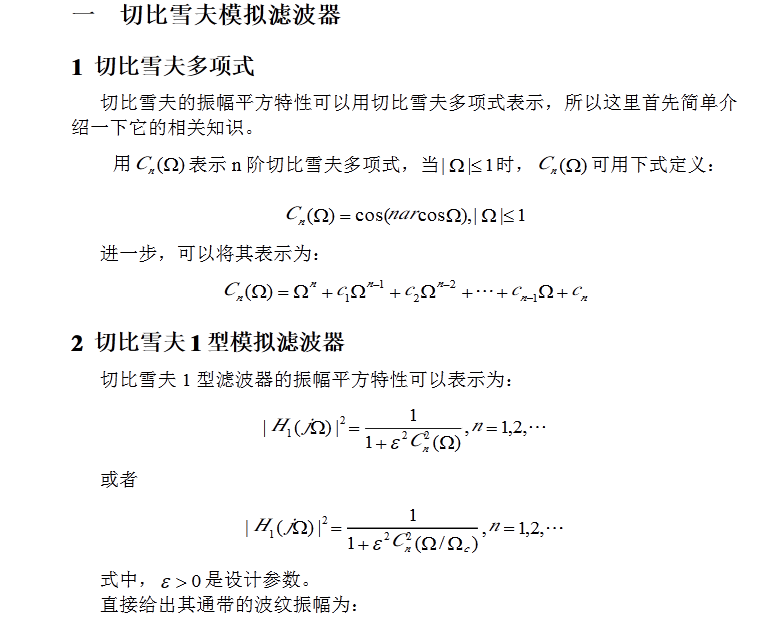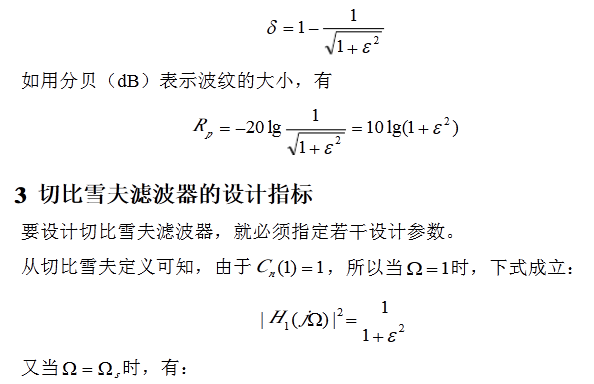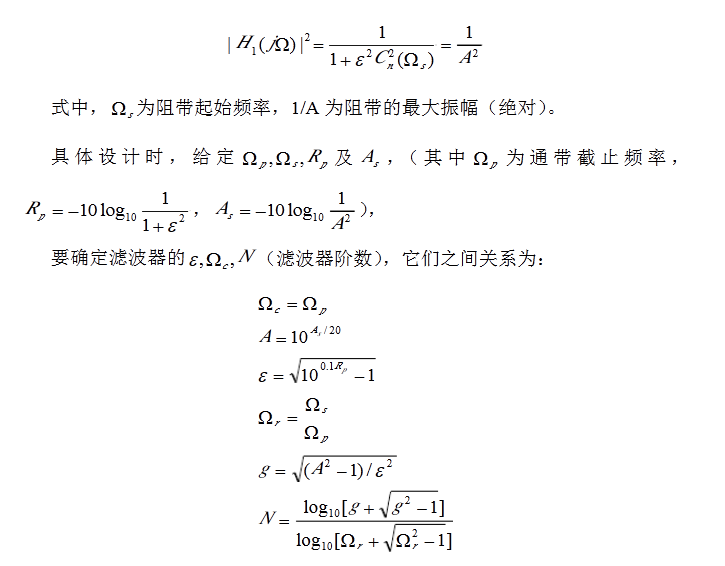带通滤波器例子

%带通滤波器使用例子

%--------------
%带通滤波器测试程序
Fsx=8000;
t=(1:Fsx)/Fsx;
ff1=10;
ff2=400;
ff3=1200;
ff4=2000;
ff5=3800;
x=50*sin(2*pi*ff1*t)+10*sin(2*pi*ff2*t)+10*sin(2*pi*ff3*t)+60*sin(2*pi*ff4*t)+60*sin(2*pi*ff5*t);
figure;
subplot(211);plot(t,x);
subplot(212);hua_fft(x,Fsx,1);
% y=filter(bz1,az1,x);
f_leftsideband=300;
f_rightsideband=3400;
f_leftcutoff=200;
f_rightcutoff=3500;
rp_sidebandreduceDB=0.1;
rs_cutoffreduceDB=30;
fs_samplefreq=8000;
y=bandp(x,f_leftsideband,f_rightsideband,f_leftcutoff,f_rightcutoff,rp_sidebandreduceDB,rs_cutoffreduceDB,fs_samplefreq);
figure;
subplot(211);plot(t,y);
subplot(212);hua_fft(y,Fsx,1);

function y=bandp(x,f1,f3,fsl,fsh,rp,rs,Fs)
%带通滤波
%使用注意事项：通带或阻带的截止频率与采样率的选取范围是不能超过采样率的一半
%即，f1,f3,fs1,fsh,的值小于 Fs/2
%x:需要带通滤波的序列
% f 1：通带左边界
% f 3：通带右边界
% fs1：衰减截止左边界
% fsh：衰变截止右边界
%rp：边带区衰减DB数设置
%rs：截止区衰减DB数设置
%FS：序列x的采样频率
% f1=300;f3=500;%通带截止频率上下限
% fsl=200;fsh=600;%阻带截止频率上下限
% rp=0.1;rs=30;%通带边衰减DB值和阻带边衰减DB值
% Fs=2000;%采样率
%
wp1=2*pi*f1/Fs;
wp3=2*pi*f3/Fs;
wsl=2*pi*fsl/Fs;
wsh=2*pi*fsh/Fs;
wp=[wp1 wp3];
ws=[wsl wsh];
%
% 设计切比雪夫滤波器；
[n,wn]=cheb1ord(ws/pi,wp/pi,rp,rs);
[bz1,az1]=cheby1(n,rp,wp/pi);
%查看设计滤波器的曲线
[h,w]=freqz(bz1,az1,256,Fs);
h=20*log10(abs(h));
figure;plot(w,h);title('所设计滤波器的通带曲线');grid on;
y=filter(bz1,az1,x);
end

function hua_fft(y,fs,style,varargin)
%当style=1,画幅值谱；当style=2,画功率谱;当style=其他的，那么花幅值谱和功率谱
%当style=1时，还可以多输入2个可选参数
%可选输入参数是用来控制需要查看的频率段的
%第一个是需要查看的频率段起点
%第二个是需要查看的频率段的终点
%其他style不具备可选输入参数，如果输入发生位置错误
nfft= 2^nextpow2(length(y));%找出大于y的个数的最大的2的指数值（自动进算最佳FFT步长nfft）
%nfft=1024;%人为设置FFT的步长nfft
y=y-mean(y);%去除直流分量
y_ft=fft(y,nfft);%对y信号进行DFT，得到频率的幅值分布
y_p=y_ft.*conj(y_ft)/nfft;%conj()函数是求y函数的共轭复数，实数的共轭复数是他本身。
y_f=fs*(0:nfft/2-1)/nfft;%?T变换后对应的频率的序列
% y_p=y_ft.*conj(y_ft)/nfft;%conj()函数是求y函数的共轭复数，实数的共轭复数是他本身。
if style==1
if nargin==3
plot(y_f,2*abs(y_ft(1:nfft/2))/length(y));%matlab的帮助里画FFT的方法
%ylabel('幅值');xlabel('频率');title('信号幅值谱');
%plot(y_f,abs(y_ft(1:nfft/2)));%论坛上画FFT的方法
else
f1=varargin{1};
fn=varargin{2};
ni=round(f1 * nfft/fs+1);
na=round(fn * nfft/fs+1);
plot(y_f(ni:na),abs(y_ft(ni:na)*2/nfft));
end

elseif style==2
plot(y_f,y_p(1:nfft/2));
%ylabel('功率谱密度');xlabel('频率');title('信号功率谱');
else
subplot(211);plot(y_f,2*abs(y_ft(1:nfft/2))/length(y));
ylabel('幅值');xlabel('频率');title('信号幅值谱');
subplot(212);plot(y_f,y_p(1:nfft/2));
ylabel('功率谱密度');xlabel('频率');title('信号功率谱');
end
end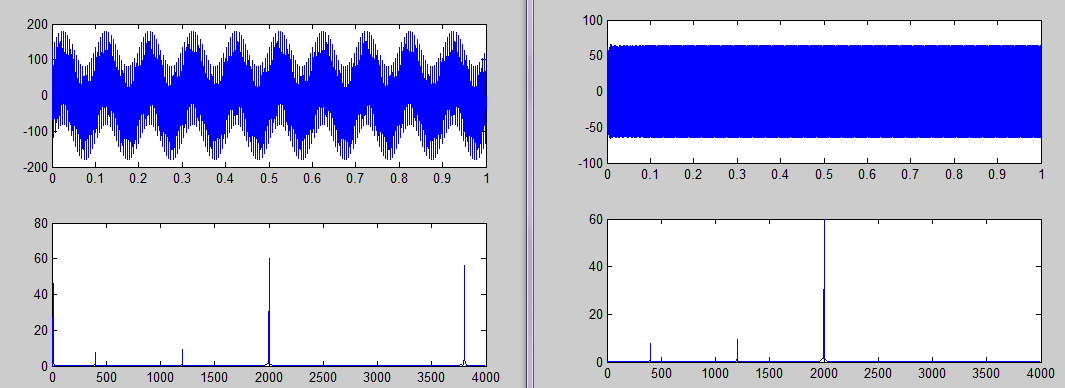az1 =

Columns 1 through 9

1.0000   -1.9928   -2.2134    5.4622    4.3571   -9.8204   -4.9934   10.3986    5.2367

Columns 10 through 18

-8.3779   -3.4684    4.2517    2.1267   -1.7583   -0.6666    0.2817    0.2634   -0.0558

Columns 19 through 21

-0.0042   -0.0444    0.0204

K>> bz1

bz1 =

Columns 1 through 9

0.0477         0   -0.4766         0    2.1446         0   -5.7189         0   10.0081

Columns 10 through 18

0  -12.0097         0   10.0081         0   -5.7189         0    2.1446         0

Columns 19 through 21

-0.4766         0    0.0477

snr
信噪比的定义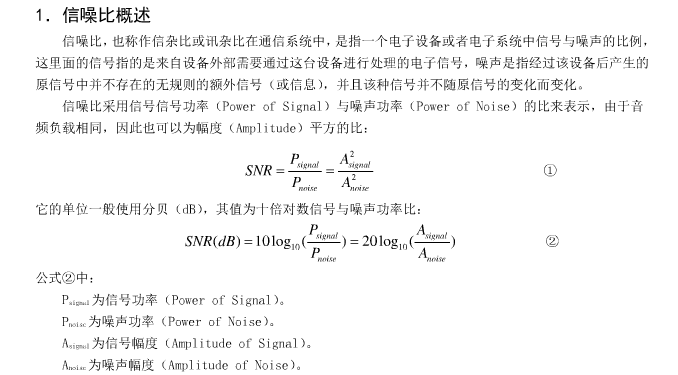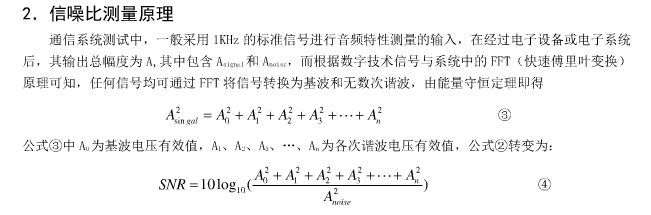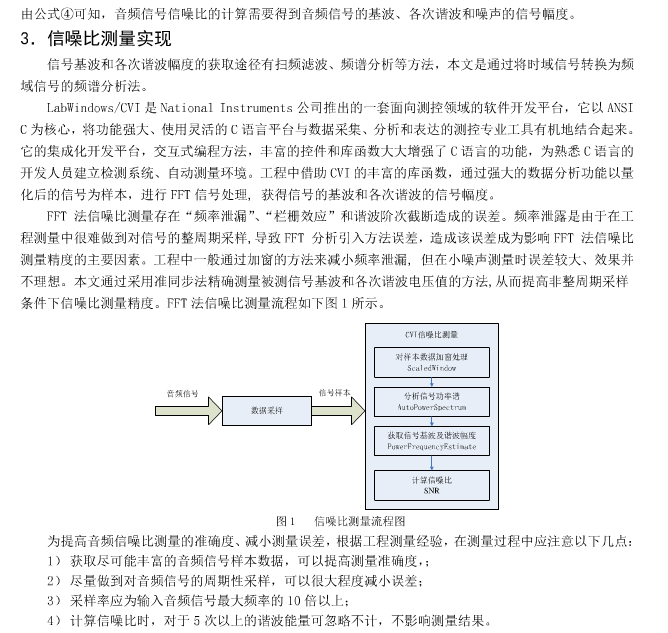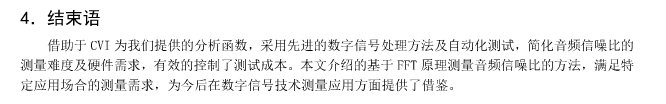MATLAB中噪声的测试

一、MATLAB中自带的高斯白噪声的两个函数

MATLAB中产生高斯白噪声非常方便，可以直接应用两个函数，一个是WGN，另一个是AWGN。WGN用于产生高斯白噪声，AWGN则用于在某一信号中加入高斯白噪声。

1. WGN：产生高斯白噪声
y = wgn(m,n,p) 产生一个m行n列的高斯白噪声的矩阵，p以dBW为单位指定输出噪声的强度。
y = wgn(m,n,p,imp) 以欧姆(Ohm)为单位指定负载阻抗。
y = wgn(m,n,p,imp,state) 重置RANDN的状态。

在数值变量后还可附加一些标志性参数：
y = wgn(…,POWERTYPE) 指定p的单位。POWERTYPE可以是'dBW', 'dBm'或'linear'。线性强度(linear power)以瓦特(Watt)为单位。
y = wgn(…,OUTPUTTYPE) 指定输出类型。OUTPUTTYPE可以是'real'或'complex'。
2. AWGN：在某一信号中加入高斯白噪声
y = awgn(x,SNR) 在信号x中加入高斯白噪声。信噪比SNR以dB为单位。x的强度假定为0dBW。如果x是复数，就加入复噪声。
y = awgn(x,SNR,SIGPOWER) 如果SIGPOWER是数值，则其代表以dBW为单位的信号强度；如果SIGPOWER为'measured'，则函数将在加入噪声之前测定信号强度。
y = awgn(x,SNR,SIGPOWER,STATE) 重置RANDN的状态。
y = awgn(…,POWERTYPE) 指定SNR和SIGPOWER的单位。POWERTYPE可以是'dB'或'linear'。如果POWERTYPE是'dB'，那么SNR以dB为单位，而SIGPOWER以dBW为单位。如果POWERTYPE是'linear'，那么SNR作为比值来度量，而SIGPOWER以瓦特为单位。

二、通过相关概念自编函数实现任意噪声的叠加及信噪比的计算

在信号处理中经常需要把噪声叠加到信号上去，在叠加噪声时往往需要满足一定的信噪比，这样产生二个问题，其一噪声是否按指定的信噪比叠加，其二怎么样检验带噪信号中信噪比满足指定的信噪比。
在MATLAB中可以用randn产生均值为0方差为1的正态分布白噪声，但在任意长度下x=randn(1,N)，x不一定是均值为0方差为1（有些小小的偏差），这样对后续的计算会产生影响。在这里提供3个函数用于按一定的信噪比把噪声叠加到信号上去，同时可检验带噪信号中信噪比。
1，把白噪声叠加到信号上去：
function [Y,NOISE] = noisegen(X,SNR)
% noisegen add white Gaussian noise to a signal.
% [Y, NOISE] = NOISEGEN(X,SNR) adds white Gaussian NOISE to X.  The SNR is in dB.
NOISE=randn(size(X));
NOISE=NOISE-mean(NOISE);
signal_power = 1/length(X)*sum(X.*X);
noise_variance = signal_power / ( 10^(SNR/10) );
NOISE=sqrt(noise_variance)/std(NOISE)*NOISE;
Y=X+NOISE;
其中X是纯信号，SNR是要求的信噪比，Y是带噪信号，NOISE是叠加在信号上的噪声。

2，把指定的噪声叠加到信号上去
有标准噪声库NOISEX-92，其中带有白噪声、办公室噪声、工厂噪声、汽车噪声、坦克噪声等等，在信号处理中往往需要把库中的噪声叠加到信号中去，而噪声的采样频率与纯信号的采样频率往往不一致，需要采样频率的校准。
% X is signal, and its sample frequency is fs;
% filepath_name is NOISE's path and name, and the SNR is signal to noise ratio in dB.
if fs1~=fs
wavin1=resample(wavin,fs,fs1);
end
nx=size(X,1);
NOISE=wavin1(1:nx);
NOISE=NOISE-mean(NOISE);
signal_power = 1/nx*sum(X.*X);
noise_variance = signal_power / ( 10^(SNR/10) );
NOISE=sqrt(noise_variance)/std(NOISE)*NOISE;
Y=X+NOISE;
其中X是纯信号，filepath_name是指定噪声文件（.wav）的路径和文件名，SNR是要求的信噪比，fs是信号X的采样频率，Y是带噪信号，NOISE是叠加在信号上的噪声。

3，检验带噪信号的信噪比
信噪比的定义为
信号能量              (纯信号)^2
SNR=-----------------=--------------------------
噪声能量        (带噪信号-纯信号)^2

function snr=SNR_singlech(I,In)
% 计算信噪比函数
% I :original signal
% In:noisy signal(ie. original signal + noise signal)
snr=0;
Ps=sum(sum((I-mean(mean(I))).^2));%signal power
Pn=sum(sum((I-In).^2));           %noise power
snr=10*log10(Ps/Pn);
其中I是纯信号，In是带噪信号，snr是信噪比

以下给出调用上函数的例子可作参考：
例一
clear all; clc; close all;
[filename,pathname]=uigetfile('*.wav','请选择语音文件：');
[Y,NOISE] = noisegen(X,10);
subplot 311; plot(X);
subplot 312; plot(NOISE);
subplot 313; plot(Y);
mn=mean(NOISE)
snr=SNR_singlech(X,Y)

例二
clear all; clc; close all;
[filename,pathname]=uigetfile('*.wav','请选择语音文件：');
[filename1,pathname1]=uigetfile('*.wav','请选择噪声文件：');
filepath_name=[pathname1 filename1];
subplot 311; plot(X);
subplot 312; plot(NOISE);
subplot 313; plot(Y);
mn=mean(NOISE)
snr=SNR_singlech(X,Y)

M文件例子

最近看了论坛中关于信噪比计算的帖子，依然困惑中，贴出来大家帮忙解答，下面是我编写的一个程序，对一个语音信号加噪去噪后求信噪比
clc
clear all
I=length(y);
[s,noise]=noisegen(y,20);
snr1=SNR_singlech(y,s)
subplot(221)
plot(y)
subplot(222)
plot(s)
yy=kalmanwhite(s,1000);
yy=yy';
subplot(223)
plot(yy)
snr2=SNR_singlech(yy,s)
定义的函数一：
function snr=SNR_singlech(I,In)
% 计算信噪比函数
% I :original signal
% In:noisy signal(ie. original signal + noise signal)
snr=0;
Ps=sum(sum((I-mean(mean(I))).^2));%signal power
Pn=sum(sum((I-In).^2));           %noise power
snr=10*log10(Ps/Pn);
定义的函数二：
function [Y,NOISE] = noisegen(X,SNR)
% noisegen add white Gaussian noise to a signal.
% [Y, NOISE] = NOISEGEN(X,SNR) adds white Gaussian NOISE to X.  The SNR is in dB.
NOISE=randn(size(X));
NOISE=NOISE-mean(NOISE);
signal_power = 1/length(X)*sum(X.*X);
noise_variance = signal_power / ( 10^(SNR/10) );
NOISE=sqrt(noise_variance)/std(NOISE)*NOISE;
Y=X+NOISE;
程序没错，但是计算出的信噪比总是变化，改变加加入的噪声信噪比，从图形上看去噪效果似乎比较好，但是算出的信噪比却相反，计算出的信噪比值总是加噪时的信噪比大于去噪后的信噪比，反复验证，没有找出原因，看论坛的帖子，困惑更多了些，这个计算出的信噪比是否是某个频率点的信噪比呢？如果是，那么要用信噪比曲线来描述整个信号求信噪比该在怎么改呢？若不是，那么错在哪啊?
希望大家给予指导下，在迷惑中等待。。。

排序算法
从数组里找前m个最大或者最小的算法

#define BNNUMMAX 16
#define BNSHIFT 4

short minindex[BNNUMMAX];
short flag_unused[SAMPLEPOINTS>>1];

#define  MAX_SHORT 65535

unsigned short getsn(unsigned short * freq,short startloop,short endloop,short flag,short nums,short shifts)
{
short i,j;
unsigned long minval;
unsigned short mini=0;

for(j=startloop;j<=endloop;j++)
{
flag_unused[j]=0;
}
for(j=0;j<nums;j++)
{
if(flag==0)
{
minval=MAX_SHORT;
}
else
{
minval = 0;
}

for(i=startloop;i<=endloop;i++)
{
if(flag_unused[i])
{
continue;
}
if(flag==0)
{
if(freq[i]<minval)
{
mini=i;
minval=freq[i];
}
}
else
{
if(freq[i]>minval)
{
mini=i;
minval=freq[i];
}
}
}
minindex[j]=mini;
flag_unused[mini]=1;
}

minval=0;
for(i=0;i<nums;i++){
print("[%d,%d]=%d",i,minindex[i],freq[minindex[i]]);
minval+=freq[minindex[i]];
}

minval = minval >>shifts;

return minval;
}
//extern unsigned short signal_val[CHANNELS];
//extern unsigned short noise_val[CHANNELS];
void getbn(unsigned short * freq)
{
unsigned short minv;
unsigned short maxv;
short start300=(3*64)/40;
short end3400=(34*64)/40;
print("---min-----");
minv = getsn(freq,start300,end3400,0,16,4);
print("---max-----");
maxv = getsn(freq,start300,end3400,1,8,3);
// 	signal_val[ch]=maxv;
// 	noise_val[ch]=minv;
}
int mbsort(unsigned short* freq,int size)
{
int i;
int j;
unsigned short temp;
for(i=0;i<size-1;i++){
for(j=i+1;j<size;j++){
if(freq[j]<freq[i]){
temp=freq[i];
freq[i]=freq[j];
freq[j]=temp;
}

}
}
for(i=0;i<size;i++){
print("[%d]=%d",i,freq[i]);
}
return 0;
}


展开全文• 惯性约束核聚变快点火实验, 要求拍瓦终端输出再压缩的高信噪比超短激光脉冲。...数值模拟了抽运激光脉冲中ASE噪声对信噪比以及对时间底座的宽度的影响, 并探讨了有利于提高超短激光脉冲信噪比的途径。
• 肿瘤内信噪比影响生理参数的研究，张洋，钱鹰，去卷积方法在医学上经常用来估计患者的生理参数，比如血流（F），血容（V），平均通过时间（MTT），毛细血管表面渗透积（PS），但�
• 讨论了大气湍流乘性噪声常用的两种概率密度函数及其对接收光强信号的影响，得到了Rytov指数与光强方差、系统接收信噪比之间的关系。最后采用不同天气条件下测得的实验数据验证了结果。研究表明：在弱湍流环境下，...
• 针对平行光栅对结构的压缩器和圆形入射光束，建立了光栅拼缝所引起的光谱剪切的数学模型，并模拟了该光谱剪切对压缩脉冲信噪比影响。与双曲正割型脉冲相比，光栅拼缝对高斯型脉冲信噪比影响较小。随着光栅拼缝...
• 结合单色频率绝对相位算法仿真分析了空间低相干干涉条纹信噪比对解调中干涉级次和绝对相位的影响，仿真结果显示，当条纹信噪比大于18 dB时，可避免干涉级次跳变问题，此时解调得到的绝对相位标准差随信噪比的增加呈...
• 在考虑光纤损耗和级联放大器的情况下,推导了调制不稳定性的产生条件和增益的普适解析表达式,分析了调制不稳定性对信噪比影响,给出了一个新的计算信噪比的表达式。并利用分立步傅里叶数值方法对非线性传输进行了...
• 采用数值模拟的方法，研究了不同脉宽比和抽运波形条件下参量荧光的演化规律及其对信噪比影响。计算结果表明，在增益一定的情况下，随着脉宽比的增大，信噪比与效率-带宽积都呈先增大后减小的趋势。存在一个最佳...
• 研究了大口径级联空间滤波器中色差造成的离焦色散与传输时间延迟对超短脉冲波形与时间信噪比影响。针对神光-5 PW高功率超短脉冲系统, 通过数值模拟, 定量分析了光束口径、色差等因素与近远场脉冲波形畸变和时间...
• 剪切率对于信噪比影响   一种获得最好SNR的办法是，先确定量化比特数对应的量化区间，在这区间内SNR不受量化比特影响，然后在这区间内寻找合适的剪切率。   参考文献 Maximizing the Transmis...


剪切率和量化比特噪声之间存在着制约关系。量化噪声对于SNR的影响剪切率对于信噪比的影响

一种获得最好SNR的办法是，先确定量化比特数对应的量化区间，在这区间内SNR不受量化比特影响，然后在这区间内寻找合适的剪切率。

参考文献
Maximizing the Transmission Performance of Adaptively Modulated Optical OFDM Signals in Multimode-Fiber Links by Optimizing Analog-to-Digital Converters

展开全文• 解释了大口径光学系统波前误差造成时间信噪比退化的原因，计算分析随机波前误差参数（误差大小、空间尺度）对聚焦时间信噪比影响，并针对不同频段的光学系统波前误差控制提出要求。明确大口径光学系统中随机波前...
高速ADC时钟jitter求解

一、模拟前端动态输入范围和有效位ENOB的关系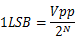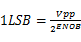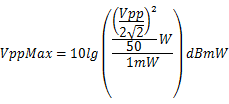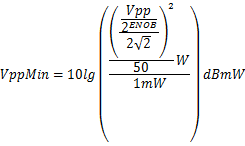模拟输入的幅度宽度：
VppMax-VppMin=6.02ENOB
2.1、SNR
SNR的定义是信号幅度均方根与噪声幅度均方根的比值。假设信号幅度均方根是S，噪声均方根是N，则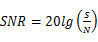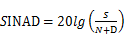2.2、THD
THD指的是基波信号的均方根值与其谐波(一般仅前5次谐波比较重要)的和方根的平均值之比。假设2次、3次、4次以上的和谐波失真分别为HD2，HD3，HDn，总谐波失真是D，则THD可以用下面公式求解：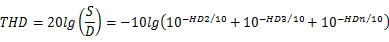信纳比和有效位数之间满足一个确定的关系：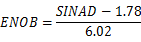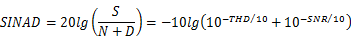三、SNR求解
3.1、量化噪声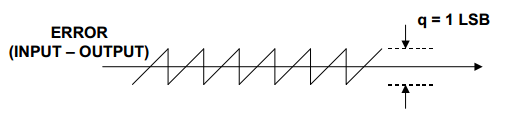量化误差引起的信噪比计算公式：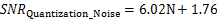3.2、热噪声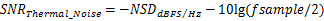NSD：noise spectral density or noise floordensity
3.3、抖动噪声
抖动噪声主要是由于时钟抖动和孔径抖动造成的。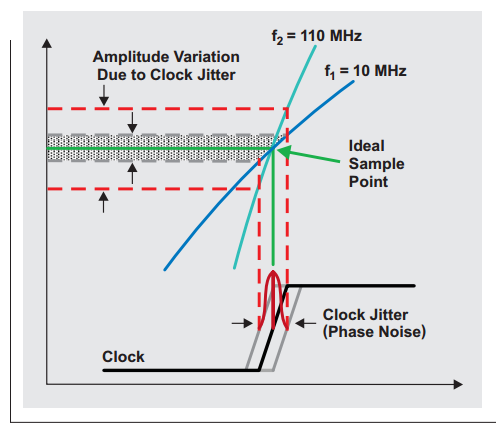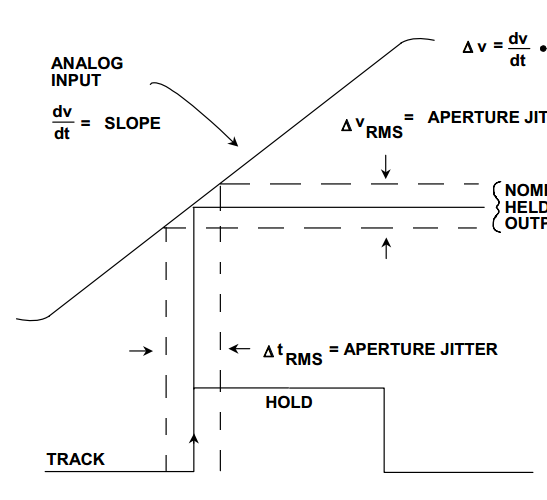上面两个图分别描述了时钟抖动和孔径抖动对采样点的影响，这两个抖动都会造成采样点的偏移，然而最后对数据处理的时候，会默认这些点都在理想位置采样的，在频域上会造成信号频率的弥散；另外一个理解方法是每一个采样点的实际采样值和理想采样值都有一定的偏差，相当于对每一个点都叠加了一个噪声。
时钟抖动引起的噪声的信噪比使用下面公式计算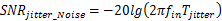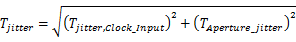从上面两个公式可以得出信噪比与采样时钟jitter成反比，与输入信号频率成反比，因此对于输入信号的频率越高，对时钟信号的jitter要求越严格。
3.4、总噪声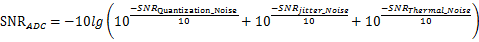四、jitter求解实例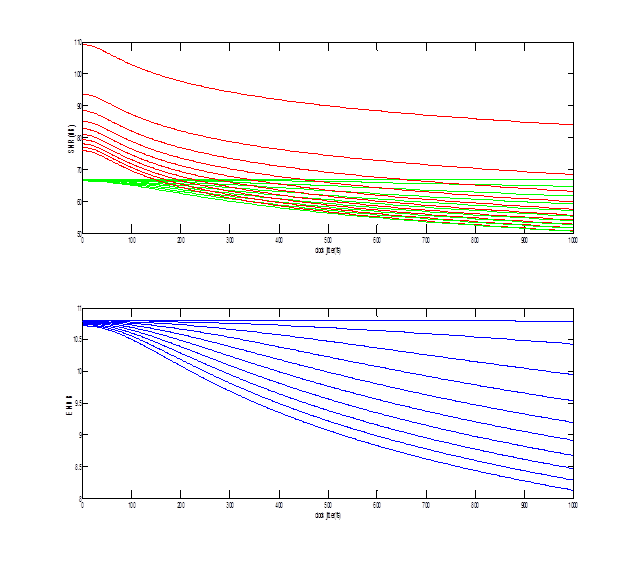第二幅图是jitter、fin、ENOB之间的关系，从上往下是不同fin对应的SNR，频率是从上依次1MHz，到501MHZ，步长50MHz。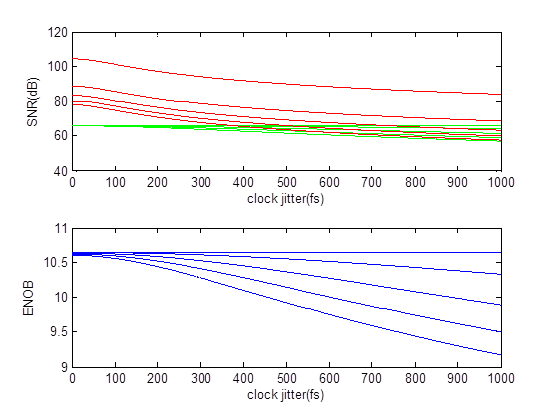第二幅图是jitter、fin、ENOB之间的关系，从上往下是不同fin对应的SNR，频率是从上依次1MHz，到251MHZ，步长50MHz。


展开全文高速ADC jitter 有效位数
• 1、热噪声为信道固有的,引起的差错是随机差错,可以通过提高信噪比来降低它对数据传输的影响。 2、冲击噪声为外界电磁干扰引起的,它是引起传输差错的最主要原因,无法通过提高信噪比来避免。 ...
通信信道的噪声主要分为两种:热噪声和冲击噪声。
1、热噪声为信道固有的,引起的差错是随机差错,可以通过提高信噪比来降低它对数据传输的影响。
2、冲击噪声为外界电磁干扰引起的,它是引起传输差错的最主要原因,无法通过提高信噪比来避免。
展开全文• 本文将探讨手机性能的一个关键方面，即手机音频播放，特别是MP3播放器到耳机的音频输出。
• 根据不同馈源接收回波信号时产生的相位差，再结合已知的目标距离，从而获取目标高度信息，针对比相测高的精度受多种因素的影响问题，着重讨论其中的一个因素信噪比对测高精度的影响，进行了仿真分析，得到了有关结论...
• 详细描述信噪比，IQ，以及灵敏度之间的关联，比较容易理解。
• 光束分束比对2μm平衡式相干探测系统信噪比影响的实验研究研究论文
• 主要研究单探测器的偏振光学相干层析系统（Polarization-sensitive optical cohrence tomography，PS-OCT）的成像信噪比，探索不同系统结构对系统信噪比影响。分析并比较使用差分探测器和非差分探测器的三种单探测...
• 信噪比估计非理想条件下，给出了一种简单的先验信息模型，使用外信息转移图分析了信噪比估计对基于线性最小均方误差的Turbo均衡系统性能的影响。所给示例表明：在一3～6dB条件下，长帧的渐进性能对估计偏差并不...
• 在QPSK调制方式下，分别研究推导了基于辅助数据的极大似然比...通过仿真比较了信噪比估计算法的性能，着重分析比较了采用的迭代次数及数据长度等参数对算法性能的影响，最终根据算法各自的特点给出了相应的适用范围。
• 随着计算机科学的发展,声学的可听化...结果表明,对于同一空间中的不同接收点的语言清晰度,早期衰变时间的影响信噪比影响显著；在语言清晰度为主要设计指标的厅堂中,应该主要控制早期衰变时间,使其不能超过1 .5 s.
• 给出了平衡式相干探测系统的一般信噪比数学模型，对该数学模型和普通单源相干探测系统的信噪比模型进行了数值仿真对比研究。仿真结果表明，当平衡式相干探测系统中的光电探测器理想匹配时，其系统的信噪比以分束比...
• 噪声抑制算法中，谱减算法用的是后验证...这个结论其实通过验证可以得出，先验信噪比影响噪声抑制的主要参数，后验信噪比是辅助参数。  那么先验信噪比与后验信噪比它们之间又有什么关系，这里做一个分析。
• ## 先验信噪比估计

千次阅读 2019-09-30 17:41:14
先验信噪比估计 很多降噪算法如维纳滤波、MMSE估计器等都依赖先验信噪比(priori SNR)信息 定义先验信噪比(priori SNR)、后验信噪比(posteriori SNR)如下 ξk(n)=E{Ak2(n)}λd(k,n)(1) \xi_{k}(n)=\frac{E\left\{A_{k...webrtc
• 这个结论其实通过验证可以得出，先验信噪比影响噪声抑制的主要参数，后验信噪比是辅助参数。   那么先验信噪比与后验信噪比它们之间又有什么关系，这里做一个分析。中间如有不清楚的地方，可以到群噪声抑制...